Courses

# Quantity Index Numbers, Business Mathematics and Statistics B Com Notes | EduRev

## B Com : Quantity Index Numbers, Business Mathematics and Statistics B Com Notes | EduRev

The document Quantity Index Numbers, Business Mathematics and Statistics B Com Notes | EduRev is a part of the B Com Course Business Mathematics and Statistics.
All you need of B Com at this link: B Com

QUANTITY INDEX NUMBERS

Just as the price index number measures the changing prices of the goods so a quantity index number measures the change in quantity/volume of the goods produced, sold or consumed. The method of construction of quantity index number are similar to the methods discussed above in the context of price index. The only difference is that the quantity index formula are obtained from the corresponding price index formula by an interchange of p by q & q by p.
Thus the following list of formulae can be derived :

Unweighted Index : Simple Aggregative Method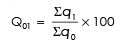Unweighted Index : Simple Average of Quantity Relative Method –

When Arithmetic Mean is used for averaging the relatives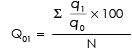– When Geometric Mean is used for averaging the relatives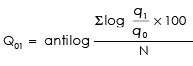Weighted Index : Simple Aggregative Method

– Laspeyres’ Method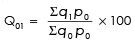– Paasche’s Method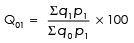– Dorbish & Bowley’s Method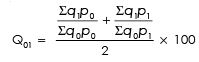– Fisher ‘ideal’ Method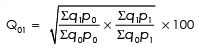– Marshall-Edgeworth Method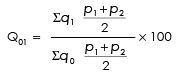— Kelly’s Method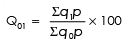Weighted Index : Weighted Average of Relative Method

– When Arithmetic Mean is used for averaging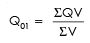where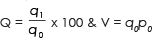– When Geometric Mean is used for averaging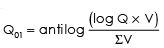Offer running on EduRev: Apply code STAYHOME200 to get INR 200 off on our premium plan EduRev Infinity!

122 videos|142 docs

,

,

,

,

,

,

,

,

,

,

,

,

,

,

,

,

,

,

,

,

,

,

,

,

;